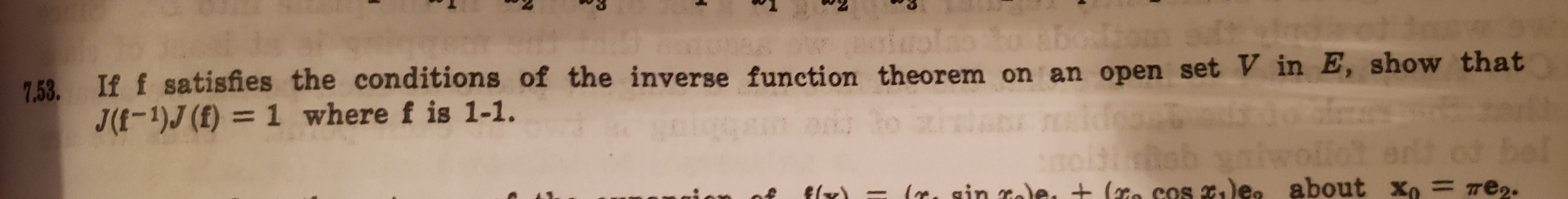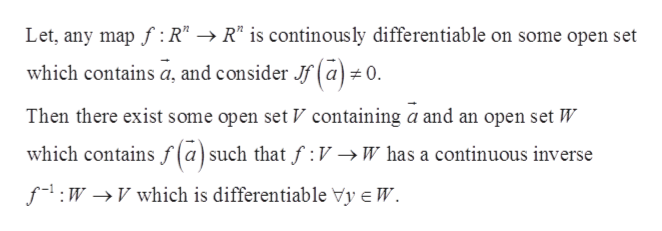# swedot7.53 If f satisfies the conditions of the inverse function theorem on an open set V in E, show thatJ(f-1)J (f) = 1 where f is 1-1.bolelt odre2.Xo14ob yLe. sin cale. + (o cos dea about

Question
13 views

Please note that f denotes a vector function defined by the set of vectors V in E (one dimensional Euclidean space).help_outlineImage Transcriptioncloseswedot 7.53 If f satisfies the conditions of the inverse function theorem on an open set V in E, show that J(f-1)J (f) = 1 where f is 1-1. bol elt od re2. Xo 14ob y Le. sin cale. + (o cos dea about fullscreen
check_circle

Step 1

Inverse function theorem-help_outlineImage TranscriptioncloseLet, any mapf R" -R" is continous ly differentiable on some open set which contains a, and consider Jf(a # 0. Then there exist some open set V containing a and an open set W which contains f (a) such that f V->W has a continuous inverse fWVwhich is differentiable Vy e W fullscreen
Step 2

Jacobi matrix satisfies composable differentiable function

Step 3

Put the values in the r...

### Want to see the full answer?

See Solution

#### Want to see this answer and more?

Solutions are written by subject experts who are available 24/7. Questions are typically answered within 1 hour.*

See Solution
*Response times may vary by subject and question.
Tagged in

### Math